# Simplification TNPSC Group 4 VAO Questions

## Simplification MCQ Questions

1.

Simplify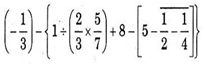Group 2 - 2018

A.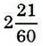B.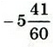C.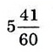D.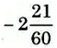B.2.

Simplify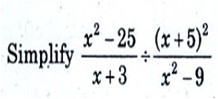Group 1 - 2019

A.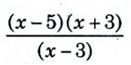B.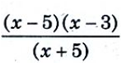C.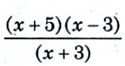D.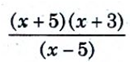B.3.

Simplify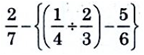Group 1 - 2019

A.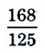B.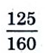C.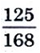D.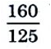C.4.

Simplify the following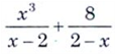Group 1 - 2019

A.

x2-2x+4

B.

x2+2x+4

C.

x2-2x-4

D.

x2+2x-4

B. x2+2x+4

5.

Simplify :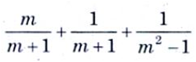Group 1 - 2019

A.

m2

B.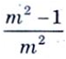C.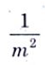D.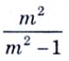D.6.

If 3a + 4b = 22, 8a - 5b = -4, then the value of (a+b)2 = ?

A.

36

B.

72

C.

25

D.

49